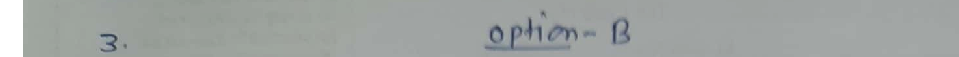Question

Linear Algebra

Determine whether the statement below is true or false Justify the answer.

Elementary row operations on an augmented matrix never change the solution set of the associated linear system.

O B. The statement is false. Interchanging two rows never changes the solution set of the associated linear system. However, scaling a row by a nonzero constant can change the solution set of that system.

the solution has been found.Ob. The statement is true. Each elementary row operation replaces a system with an equivalent system

The statement is false. Interchanging two rows never changes the solution set of the associated linear system. However, replacing one row by the sum of itself and a multiple of another row can change the solution set of that system.The statVerified### Question 45665Linear Algebra

\text { 4] Find } g(0)-g(9)+g(2) \text {, if }
g(x)=\left\{\begin{aligned} \frac{x+1}{2}, & \text { if } x \text { is odd } \\ \frac{x}{2}, & \text { if } x \text { is even } \end{aligned}\right.

### Question 45664Linear Algebra

\text { 3] Find } f(4)-f(2)+f(3) \text {, if }
f(x)=\left\{\begin{array}{ll} \frac{x+1}{2}, & \text { if } x \text { is odd } \\ \frac{x}{4}, & \text { if } x \text { is even } \end{array}\right.

### Question 45663Linear Algebra

2] Find the Domains of the following functions:
\text { a) } f(x)=\sqrt{15-5 x}
f(x)=\frac{x^{2}-2 x+1}{x^{2}-4 x-21}
f(x)=\frac{x^{2}-2 x+1}{\sqrt{16-2 x}}

### Question 45662Linear Algebra

a) Give the definition of a rational function. [5 pts]
b) Give an example of a polynomial function of degree 3. [5 pts]
c) Can a constant function be a polynomial and a rational function at the same time? Explain your answer. [5 pts]
d) Give an example of a non-polynomial function and explain why not apolynomial function. [10 pts)

### Question 45333Linear Algebra

5) Ве,
\begin{array}{c} f: \mathbb{R} \rightarrow \mathbb{R} \\ f(x)=\left\{\begin{array}{ll} x^{2}-3 \cos (\pi x) & x<0 \\ x-4 e^{-2 x} & x \geq 0 . \end{array}\right. \end{array}
Calculate
\int_{-1}^{2} f(x) d x
Presenting the result in simplified form

### Question 45332Linear Algebra

4) Be f: R - Ra function differentiable in R such your derivative f', has in maximum a real zero.
Prove that the equation f(x)=0 has in maximum 2 real square

### Question 45331Linear Algebra

3) Take in consideration the following function
f(x)=\left\{\begin{array}{ll} f: \mathbb{R} \rightarrow \mathbb{R} & \\ \frac{x^{2}-4 x+\cos (\sin (x)),}{x^{4}+4 x^{2}+1}, & x \leq 0 \end{array}\right.
a) Show that the function f is continuous in R+ and in R- but discontinuous in the point X
b) Say justifying, if f is differenciable in X=0

### Question 45330Linear Algebra

2) Prove by definition that
\lim _{x \rightarrow 0} x^{2} \cos \left(e^{x}\right)=0

### Question 45329Linear Algebra

1) Calculate the following limit:
\lim _{x \rightarrow+\infty} \frac{x^{2}\left(e^{-3 x}+1\right)+x \cos (5 x)}{x^{2}+7 x+1}

### Question 45305Linear Algebra

7. Consider the following problem.
Maximize Z = 6X1 + 8X2
5X1 + 2X2 <=20
X1 + 2X2 <=10
X1, X2 >= 0
a. Construct the dual problem for this primal problem
b. Solve both the primal problem and the dual problem graphically.Identify the CPF (Corner-Point Feasible) solutions and corner -pointinfeasible solutions for both problems. Calculate the objectivefunction values for all these solutions.
c. Construct a table listing the complementary basic solutions for theseproblems.
d. Work through the simplex method step by step to solve the primal problem. After each iteration, identify the basic feasible (BF) solution for this problem and the complementary basic solution for the dual problem. Also identify the corresponding corner-point solutions.

### Submit query